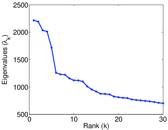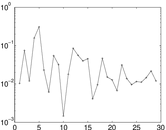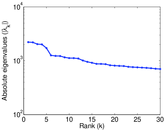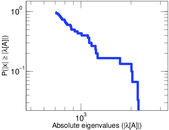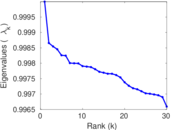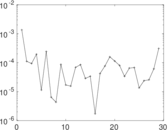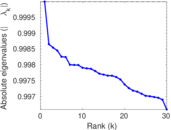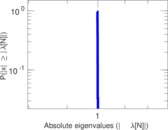This is a bipartite network consisting of Twitter users and tags they mentioned in their postings. Left nodes represent users and right nodes represent tags. An edge shows that a tag was used by a user in a tweet.

 Code `Wut` Internal name `munmun_twitterex_ut` Name Twitter user–tag Data source http://www.public.asu.edu/~mdechoud/datasets.html AvailabilityDataset is available for download Consistency checkDataset passed all tests Category Interaction network Dataset timestamp 2010 Node meaning User, hashtag Edge meaning Usage Network formatBipartite, undirected Edge typeUnweighted, multiple edges Temporal dataEdges are annotated with timestamps Snapshot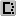Is a snapshot and likely to not contain all data

## Statistics

 Size n = 705,632 Left size n1 = 175,214 Right size n2 = 530,418 Volume m = 4,664,605 Unique edge count m̿ = 1,890,661 Wedge count s = 1,006,768,611 Claw count z = 3,620,991,361,242 Cross count x = 14,011,028,889,674,202 Square count q = 206,508,691 4-Tour count T4 = 5,682,999,878 Maximum degree dmax = 90,362 Maximum left degree d1max = 2,431 Maximum right degree d2max = 90,362 Average degree d = 13.221 1 Average left degree d1 = 26.622 3 Average right degree d2 = 8.794 21 Fill p = 2.034 35 × 10−5 Average edge multiplicity m̃ = 2.467 18 Size of LCC N = 690,906 Diameter δ = 16 50-Percentile effective diameter δ0.5 = 5.022 02 90-Percentile effective diameter δ0.9 = 5.893 74 Median distance δM = 6 Mean distance δm = 5.319 70 Gini coefficient G = 0.842 861 Balanced inequality ratio P = 0.149 222 Left balanced inequality ratio P1 = 0.236 392 Right balanced inequality ratio P2 = 0.135 109 Relative edge distribution entropy Her = 0.882 745 Power law exponent γ = 2.460 48 Tail power law exponent γt = 2.601 00 Degree assortativity ρ = −0.098 655 9 Degree assortativity p-value pρ = 0.000 00 Spectral norm α = 2,216.48 Algebraic connectivity a = 0.003 077 89 Spectral separation |λ1[A] / λ2[A]| = 1.010 44 Controllability C = 437,932 Relative controllability Cr = 0.620 624

## Plots

### Fruchterman–Reingold graph drawing### Degree distribution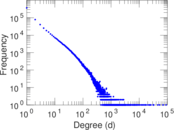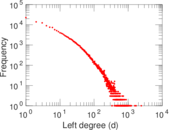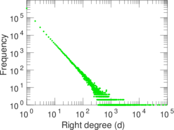### Cumulative degree distribution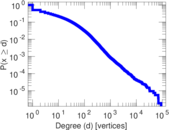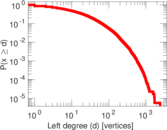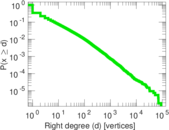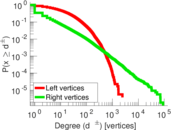### Lorenz curve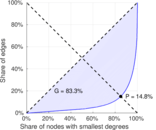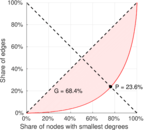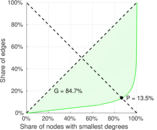### Spectral distribution of the adjacency matrix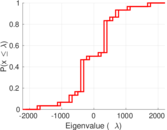### Spectral distribution of the normalized adjacency matrix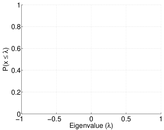### Spectral distribution of the Laplacian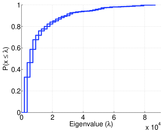### Spectral graph drawing based on the adjacency matrix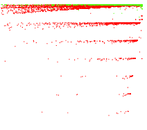### Spectral graph drawing based on the Laplacian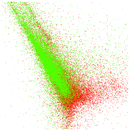### Spectral graph drawing based on the normalized adjacency matrix### Degree assortativity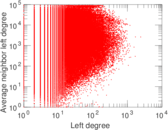### Zipf plot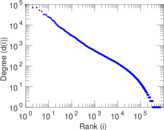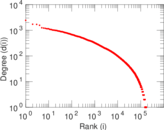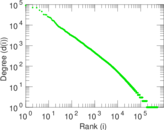### Hop distribution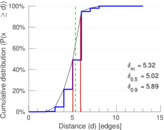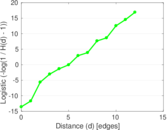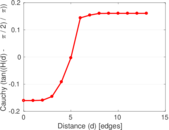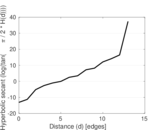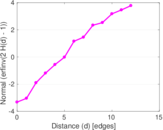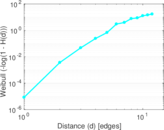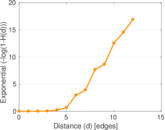### Delaunay graph drawing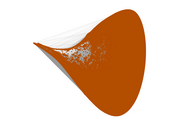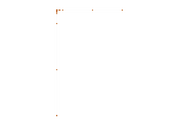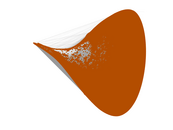### Edge weight/multiplicity distribution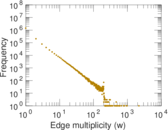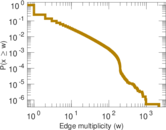### Temporal distribution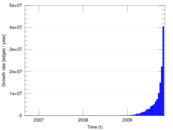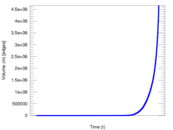### Diameter/density evolution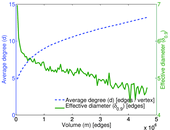### Inter-event distribution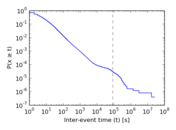### Node-level inter-event distribution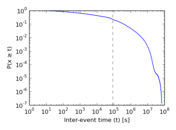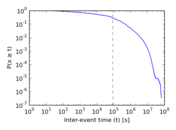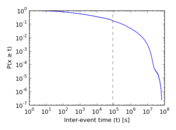### Matrix decompositions plots